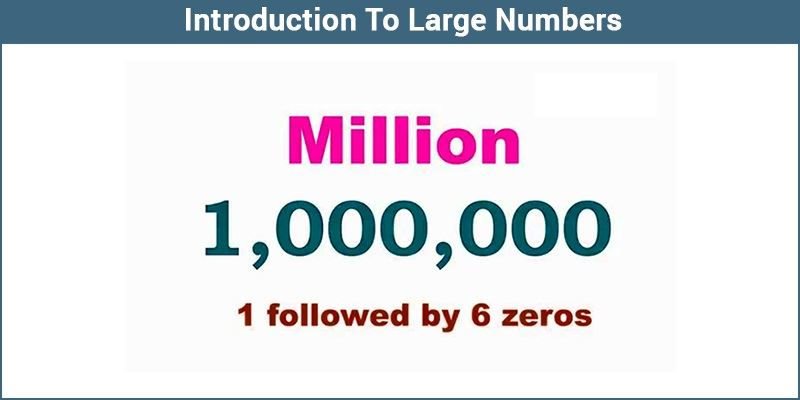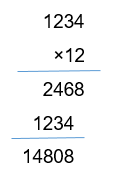# Introduction to Large Numbers

Large numbers are technically described as numbers bigger than what is used in daily life. In the number system, the numbers which are ordinarily bigger or greater than the other numbers are called large numbers. For example, 1 lakh, 1 million, 1 billion, etc. are big numbers which we do not use in daily life. These big numbers are basically denoted in standard form.

The positive large numbers are used to represent the population of the country or the amount in a bank account.## What are the Large Numbers?

Now that the root of the fear has been uncovered, it should get easier to tackle the problem. Since fear of large numbers stems from unfamiliarity, we shall make it familiar. We are well aware that numbers are separated into groups: the ones, tens, hundreds, thousands, millions, and so on. Each group is home to three subdivisions: ones, tens, and hundreds. When reading or writing, a large number begins at the left with the largest group and proceeds to the right.

For instance, take 8,685 is read as eight thousand, six hundred, and eighty-five. The first one must be clear with this. It begins with the ones: ones, tens and hundreds being the subdivisions, followed by thousands, millions, and billions and so on, with their own three subdivisions. How would you read 10,876,432? It would go ten million, eight-hundred seventy-six thousand, and four hundred and thirty-two.

The very large numbers are somewhat like this:

1,000,000,000 are “one billion”.

1,000,000,000,000 are “one thousand billion”.

1,000,000,000,000,000 are “one million billion”.

1,000,000,000,000,000,000 are “one billion billion”.

1,000,000,000,000,000,000,000 are “a thousand billion billion” and so on…

When a number is made of more than 12 figures, you say first the number of billions, then the numbers of millions, then the numbers of thousands, then the hundreds, tens, and units. The more you practice, the more the fear will subside. With normal numbers, it is possible to apply the arithmetic operations on large numbers, such as:

• Subtraction of Large Numbers
• Multiplication of Large Numbers
• Division of Large Numbers

### Addition and Subtraction of Large Numbers

In addition, if you want to add large numbers, you can stack the list of numbers on top of each other you want to add so that one’s digit lines up in a column, ten’s digit lines up in another column, etc. In this process, you can add a column by column from the right side. It means that start the addition process from the one’s column, ten’s column, a hundred’s column, and so on. This process is known as column addition. Similarly, for the subtraction of large numbers, the process is the same. Instead of addition, you do the subtraction process and it is known as column subtraction.

### Multiplication of Large Numbers

To multiply large numbers, write down the numbers on top of each other, and align the numbers. In case if there are any decimal numbers, don’t worry about the decimal values; just line up the given numbers. Let’s take the 1’s place of the bottom number and multiply it with the top number. Then you will get one result. Then, take the tens place of the bottom number and multiply it with the top numbers. Here, you should write your result in the tens place in the next line of the resultant area. Now add both the result to get the product of those numbers.

For example, Multiply 1234 by 12### Division of Large Numbers

To divide large numbers, the process called the long division method is used. Unlike other operations, the division operation is performed from left to right. In this process, for each digit in the dividend, you should finish the complete cycle of division, subtraction and multiplication operations.

## Video Lesson on Numbers## Frequently Asked Questions – FAQs

Q1

### What do we call the number 100000 in words?

The number 100000 in words is One lakh
Q2

### The smallest 5-digit number is?

The smallest 5-digit number is 10000
Q3

### The largest 5-digit number is?

The largest number 5-digit number is 99999
Q4

### What is the sum of the smallest 5-digit number and the largest 4-digit number?

The smallest 5-digit number is 10000
The largest 4-digit number is 9999
The sum of the smallest 5-digit number and the largest 4-digit number is
9999+10000=19999
Q5

### What do we call the number 999999 in words?

The number 999999 in words is Nine lakhs ninety-nine thousand nine hundred and ninety-nine